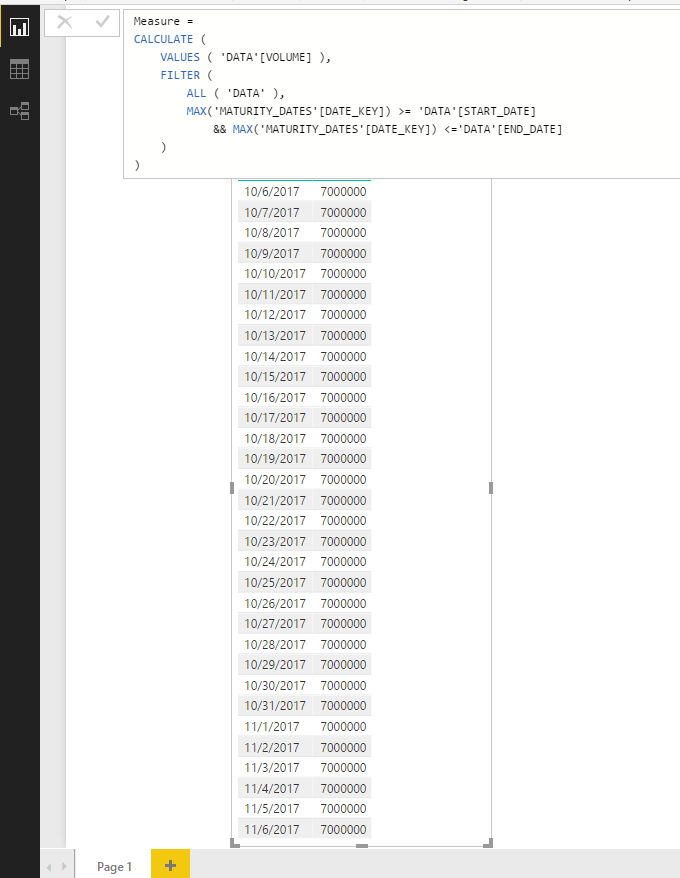cancel
Showing results for
Did you mean:Helper II

## Show same measure value for a specified range

Hi there,

I have a data table with one row.  A Transaction which has a lifetime of one month, with a value of 7000000.

 DEAL_NUMBER START_DATE END_DATE VOLUME Deal 1 10/6/2017 11/6/2017 7000000

Moreover, two date/lookup tables that are connect via relationshipsPutting MONTH and DAY from the lookup table "Maturity dates" on the rows, and a measure SUM(VOLUME) on values section of a pivot table thie following is the result.So far so good. But what I WANT to do, is modify the mesaure so the same value of 7000000 is shown for all the dates between 10/6 and 11/6.

The below image shows the desired output (sketched by me).I have tried using calculate but not succeeded.  Along the lines of...

```NewMeasure:=
CALCULATE(
[Volume]
;FILTER(
ALL(MATURITY_DATES)
;MATURITY_DATES[DATE_KEY]>= MAX(DATA[START_DATE])
;MATURITY_DATES[DATE_KEY] <= MAX(DATA[END_DATE])
)
)```

Do you spot the error in my thinking?

Cheers and kind regards

2 ACCEPTED SOLUTIONSCommunity Support

Hi @chefe

@Zubair_Muhammad's logic is right, you need to modify it slightly:

Measure =
CALCULATE (
VALUES ( 'DATA'[VOLUME] ),
FILTER (
ALL ( 'DATA' ),
MAX('MATURITY_DATES'[DATE_KEY]) >= 'DATA'[START_DATE]
&& MAX('MATURITY_DATES'[DATE_KEY]) <='DATA'[END_DATE]
)
)Best Regards,
Qiuyun Yu

Community Support Team _ Qiuyun Yu
If this post helps, then please consider Accept it as the solution to help the other members find it more quickly.Super User

Hi @chefe,

This is my calculated field formula

`=if(HASONEVALUE('Calendar'[Date]),SUM(DATA[VOLUME]),BLANK())`

Regards,
Ashish Mathur
http://www.ashishmathur.com
3 REPLIES 3Super User

Hi @chefe,

This is my calculated field formula

`=if(HASONEVALUE('Calendar'[Date]),SUM(DATA[VOLUME]),BLANK())`

Regards,
Ashish Mathur
http://www.ashishmathur.comCommunity Support

Hi @chefe

@Zubair_Muhammad's logic is right, you need to modify it slightly:

Measure =
CALCULATE (
VALUES ( 'DATA'[VOLUME] ),
FILTER (
ALL ( 'DATA' ),
MAX('MATURITY_DATES'[DATE_KEY]) >= 'DATA'[START_DATE]
&& MAX('MATURITY_DATES'[DATE_KEY]) <='DATA'[END_DATE]
)
)Best Regards,
Qiuyun Yu

Community Support Team _ Qiuyun Yu
If this post helps, then please consider Accept it as the solution to help the other members find it more quickly.Community Champion

Hi @chefe

Try this

```NewMeasure :=
CALCULATE (
VALUES ( DATA[Volume] ),
FILTER (
ALL ( DATA ),
MATURITY_DATES[DATE_KEY] >= DATA[START_DATE]
&& MATURITY_DATES[DATE_KEY] <= DATA[END_DATE]
)
)```

Regards
Zubair